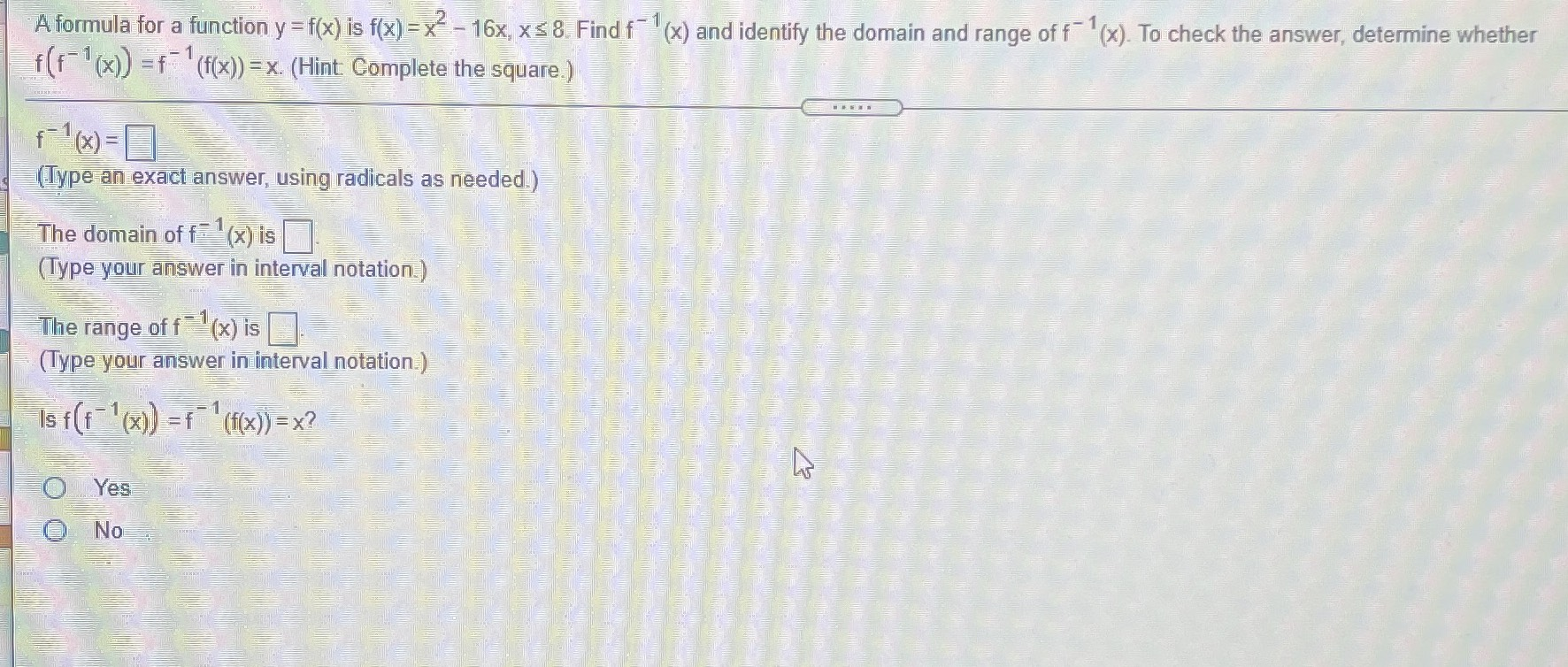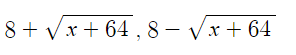### ¿Todavía tienes preguntas de matemáticas?

Pregunte a nuestros tutores expertos
Algebra
PreguntaA formula for a function $$y = f ( x )$$ is $$f ( x ) = x ^ { 2 } - 16 x , x \leq 8 .$$ Find $$f ^ { - 1 } ( x )$$ and identify the domain and range of $$f ^ { - 1 } ( x )$$ . To check the answer, determine whether

$$f ( f ^ { - 1 } ( x ) ) = f ^ { - 1 } ( f ( x ) ) = x$$ . (Hint Complete the square.)

$$f ^ { - 1 } ( x ) = \square$$

The domain of $$f ^ { - 1 } ( x )$$ is $$\square$$ .

The range of $$f ^ { - 1 } ( x )$$ is $$\square$$

Is $$f ( f ^ { - 1 } ( x ) ) = f ^ { - 1 } ( f ( x ) ) = x$$ ?

Yes

No$$x\ge - 64$$